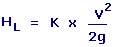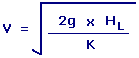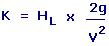Minor Losses Equation Calculators Enter value, select unit and click on calculate. Result will be displayed.

Calculate Velocity
Calculate Loss Coefficient

 Web www.calculatoredge.comHL = Head Loss K = Loss Coefficient V = Flow Velocity g = Acceleration Of Gravity
 Enter your values: Loss Coefficient: Velocity: Foot / Second Meter / Second Centimeter / Hour Centimeter / Second Foot / Day Inch / Hour Inch / Second Kilometer / Second Knot Meter / Day Mile / Hour Mile / Second Millimeter / Second Yard / Second Acceleration Of Gravity: Foot / Second^2 Meter / Second^2 Centimeter / Second^2 Inch / Second^2 Kilometer / Second^2 Mile / Second^2 Millimeter / Second^2 Yard / Second^2 Result: Head Loss: Foot

Calculate VelocityV = Flow Velocity HL = Head Loss g = Acceleration Of Gravity K = Loss Coefficient
 Enter your values: Loss Coefficient: Head Loss: Foot Meter Attometer Centimeter Dekameter Decimeter Exameter Femtometer Hectometer Inch Kilometer Megameter Micrometer Mile Millimeter Nanometer Petameter Picometer Yard Acceleration Of Gravity: Foot / Second^2 Meter / Second^2 Centimeter / Second^2 Inch / Second^2 Kilometer / Second^2 Mile / Second^2 Millimeter / Second^2 Yard / Second^2 Result: Velocity: Foot/Second

Calculate Loss CoefficientK = Loss Coefficient HL = Head Loss g = Acceleration Of Gravity V = Flow Velocity
 Enter your values: Velocity: Foot / Second Meter / Second Centimeter / Hour Centimeter / Second Foot / Day Inch / Hour Inch / Second Kilometer / Second Knot Meter / Day Mile / Hour Mile / Second Millimeter / Second Yard / Second Head Loss: Foot Meter Attometer Centimeter Dekameter Decimeter Exameter Femtometer Hectometer Inch Kilometer Megameter Micrometer Mile Millimeter Nanometer Petameter Picometer Yard Acceleration Of Gravity: Foot / Second^2 Meter / Second^2 Centimeter / Second^2 Inch / Second^2 Kilometer / Second^2 Mile / Second^2 Millimeter / Second^2 Yard / Second^2 Result: Loss Coefficient:LINKSDISCLAIMERCONTACT US